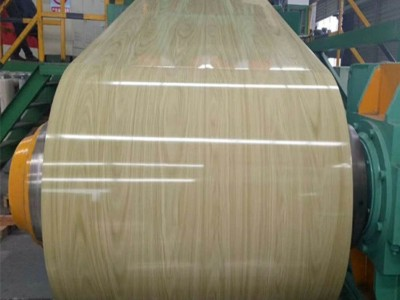# ts280gd高强度彩涂板卷的重量和价格怎么计算你知道吗？

## ts280gd高强度彩涂板卷的重量和价格怎么计算你知道吗？A、 净重是指货物的重量。根据国际惯例，合同中对重量的计算没有其他规定的，以净重计量

·实际皮重，即每个包装的重量
·平均重量。当包装相对均匀时，可以从所有商品中取出一定数量的包装并称重，计算出每个包装的平均重量
·定制皮重。适用于标准化包装。包装的重量是众所周知的，不需要称重
·约定皮重。双方事先约定的包装重量。B、 对于干含水量不稳定的货物，如羊毛、生丝、棉花等，为了准确计算货物重量，国际上常用的按规定重量计算的方法是测量货物的实际回潮率（含水量）来计算货物的净重，公式如下：一般数量=[货物的实际重量/（10实际退货率）]x（10固定退货率）=净重商品x（10恒定回报率）C.理论重量

Do you know how to calculate the weight of the color coated coil? Now Xiaobian will tell you. Generally, the formula is: the thickness of color plate (m m) × width (m) × total meter (m) × 7.85 = (kg) 7.85 is the density of iron, and the weight of iron per cubic meter is 7.85 tons. 1531275862.jpg
In the international trade, there are many goods measured by weight. According to the general business habits, there are several ways to calculate the weight:
A. Net weight refers to the weight of the goods. According to international practice, if there is no other provision for the calculation of weight in the contract, it shall be measured by net weight
Some commodities can only be weighed after being packed, and the weight obtained is gross weight. For commodities with lower value, gross weight can be stipulated in the contract, that is, gross for net. If net weight is needed, the weight of packages must be subtracted from gross weight, that is, tare weight. There are several ways to calculate tare weight:
·Actual tare weight, i.e. weight of each package
·Average weight. When the packages are relatively uniform, a certain number of packages can be taken from all commodities, weighed, and the average weight of each package can be calculated
·Custom tare weight. Suitable for standardized packaging. The weight of packaging is well known and does not need to be weighed
·Agreed tare weight. The packing weight agreed by both parties in advance. B. for goods with unstable dry moisture content, such as wool, raw silk, cotton, etc., in order to accurately calculate the weight of such goods, the internationally commonly used method of calculating according to the conditioned weight is to measure the actual moisture regain (moisture content) of the goods to calculate the clean weight of the goods, The formula is as follows: common quantity = [actual weight of commodity / (10 actual return rate)] x (10 constant return rate) = net weight of commodity x (10 constant return rate) C. theoretical weight
You use 0.35 × 7.85 (density of iron) × market price, and then divide by 1000 to get the price per meter.

【相关推荐】

400-060-9166
• 400电话

400-060-9166